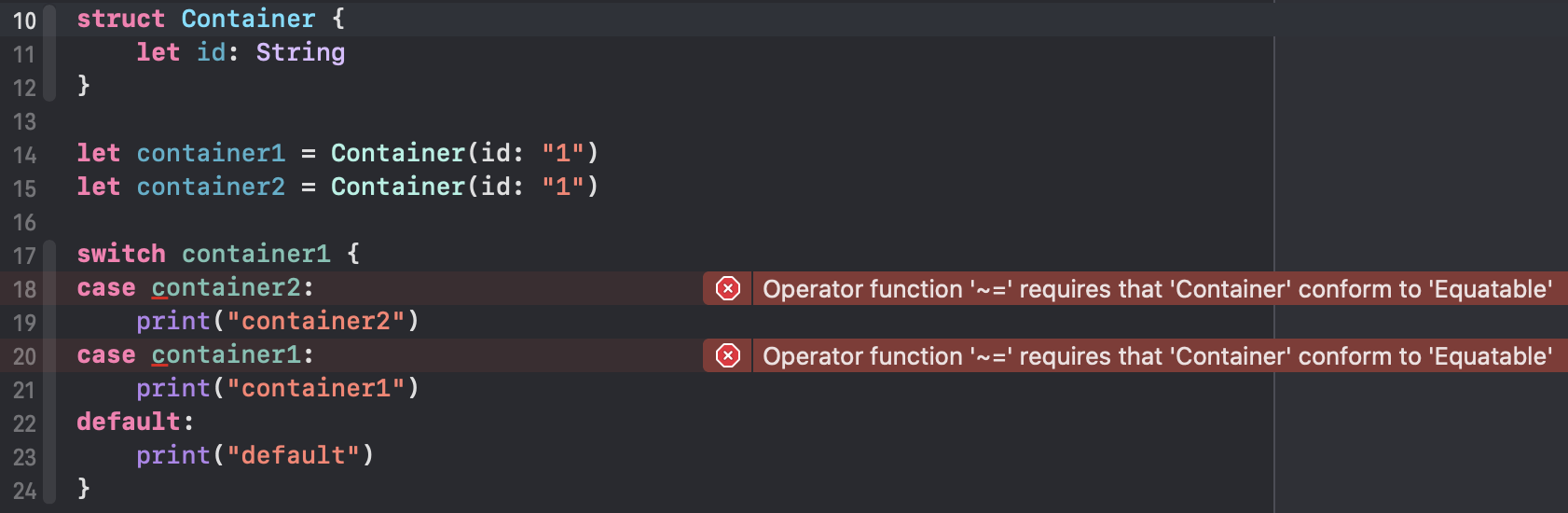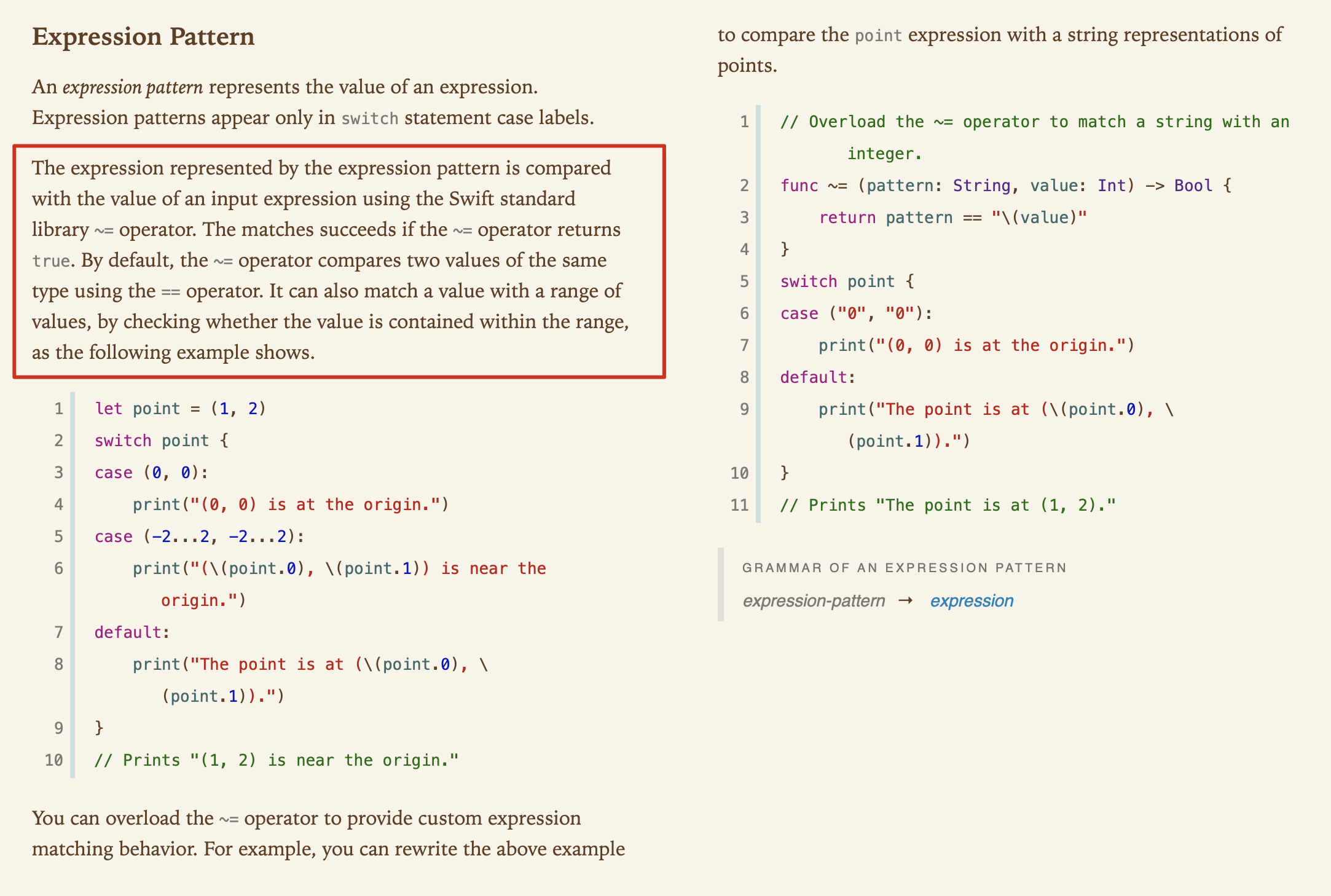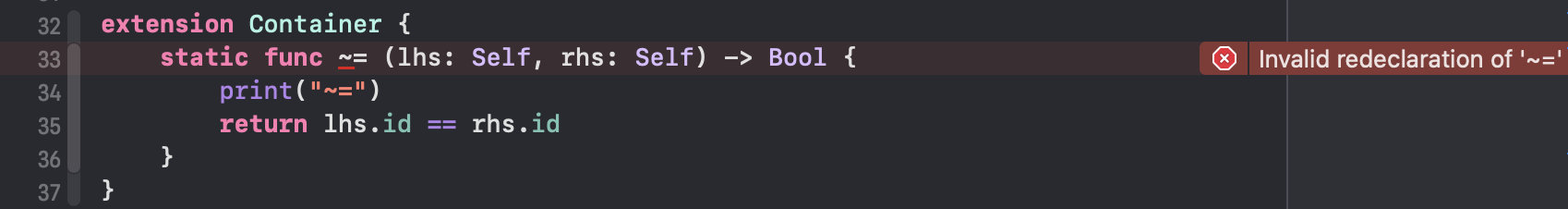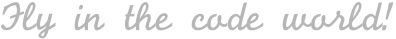## 内容概览

• 前言
• 使用 `switch` 匹配自定义类型的实例
• 遵循 `Equatable` 协议
• 自定义 `~=` 运算符
• `~=` 运算符
• `~=` 运算符与 `==` 运算符
• 尽量不要自定义 `~=` 运算符
• 总结

## 前言

`switch` 是我们在使用 Swift 进行开发时经常用到的语句。基于 `switch`，我们可以很惬意地匹配枚举类型实例。然而，可能有一些朋友像我一样不明白 switch 的匹配原理。

## 使用 switch 匹配自定义类型的实例

``````struct Container {
let id: String
}

let container1 = Container(id: "1")
let container2 = Container(id: "2")

switch container1 {
case container2:
print("container2")
case container1:
print("container1")
default:
print("default")
}
``````• `switch` 匹配基于 `~=` 运算符；
• `switch` 中进行匹配的值，其类型需要遵循 `Equatable`

## 遵循 Equatable 协议

``````struct Container: Equatable { // 遵循 Equatable 协议
let id: String
}

let container1 = Container(id: "1")
let container2 = Container(id: "2")

switch container1 {
case container2:
print("container2")
case container1:
print("container1")
default:
print("default")
}
``````

``````container1
``````

``````final class Container: Equatable {
let id: String

static func == (lhs: Container, rhs: Container) -> Bool {
return lhs.id == rhs.id
}

init(id: String) {
self.id = id
}
}
``````

Ficow 推荐您采用这种方式来解决 switch 匹配问题，为什么呢？ 答案在后文中，请您继续阅读~

## 自定义 `~=` 运算符

#### `~=` 运算符``````print(200...299 ~= 404)
``````

``````struct Container {
let id: String

static func ~= (lhs: Self, rhs: Self) -> Bool {
return lhs.id == rhs.id
}
}
``````

``````func ~= (lhs: Container, rhs: Container) -> Bool {
return lhs.id == rhs.id
}

struct Container {
let id: String
}
``````

#### `~=` 运算符与 `==` 运算符

``````struct Container: Equatable {
let id: String

static func ~= (lhs: Self, rhs: Self) -> Bool {
print("~=")
return lhs.id == rhs.id
}

static func == (lhs: Self, rhs: Self) -> Bool {
print("==")
return lhs.id == rhs.id
}
}

let container1 = Container(id: "1")
let container2 = Container(id: "2")

switch container1 {
case container2:
print("container2")
case container1:
print("container1")
default:
print("default")
}
``````

``````~=
~=
container1
``````

#### 尽量不要自定义 `~=` 运算符

``````// framework A
public struct Container: Equatable {
let id: String

public static func == (lhs: Self, rhs: Self) -> Bool {
print("==")
return lhs.id == rhs.id
}
}

// framework B
let container1 = Container(id: "1")
let container2 = Container(id: "2")

switch container1 {
case container2:
print("container2")
case container1:
print("container1")
default:
print("default")
}
``````

``````extension Container {
static func ~= (lhs: Self, rhs: Self) -> Bool {
print("~=")
return lhs.id == rhs.id
}
}
``````## 总结

Swift - Expression Pattern

#### 评论区(期待你的留言)